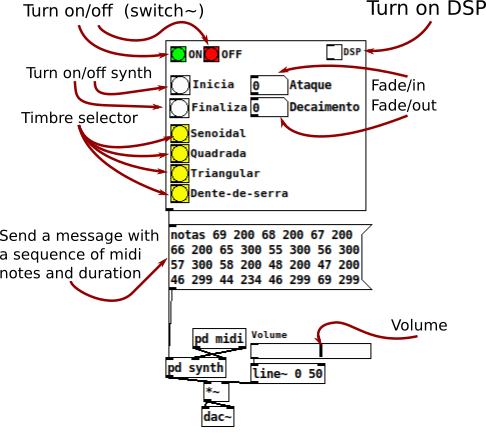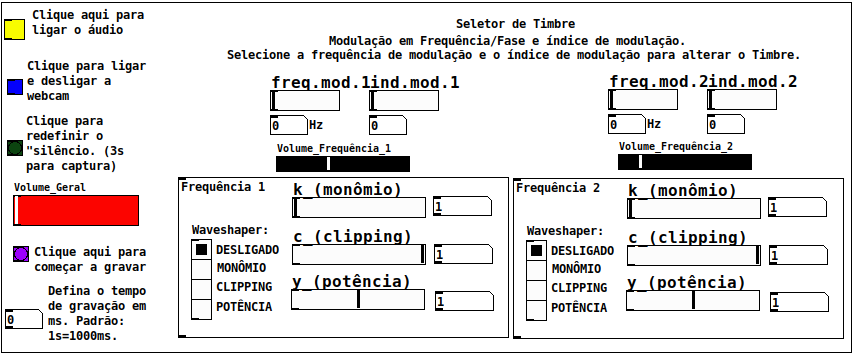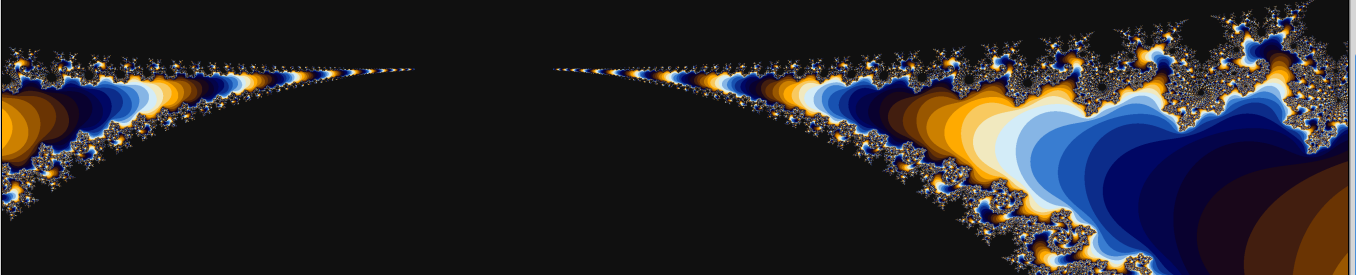### Multi-Timbre Synthesizer

This synthesizer was built as a Program Exercise (EP) to Computer Music class, from the Institute of Mathematics and Statistics of the University of São Paulo / BR, by professor Dr. Marcelo Queiroz.Miller S. Puckette's "vanilla" distribution of Pd
Last release: Pure Data 0.51-3
Released 20/11/2020
Required extensions: Zexy

If you have a synth keyboard, plug it into you computer and follow this steps.
Else if, you don't have a keyboard, see this instructions.
Else if, you want to build a QWERTY synth keybord, see this Youtube video from LWM Music channel.

##### Source code### Musicam - Webcam based digital instrument

#### Digital instrument based on oscilator's frequency manipulation using Webcam images##### Source code##### Required extensions

Windows and MAC users: If you want to run this patch quickly, you can download the DMG packages or the EXE installer of Purr-Data HERE.

##### Performances
By Claudinei Sevegnani (click on images to see)### Mandelbrot Fractal

#### Performance test using different parallel configurations.##### Source codeThis work contains some of programs-exercises of class MAC5742 - Introduction to Concurrent, Parallel and Distributed Programming (2020) - Institute of Mathematics and Statistics, São Paulo University (IME-USP).

##### Authors

Carlos Eduardo Leal de Castro (me)
Felipe de Lima Peressim
José Luiz Maciel Pimenta
Luis Ricardo Manrique
Rafael Fernandes Alencar

##### Description

The Mandelbrot Set is the set of all complex points $$\mathbb{C}$$ such that the sequence $$\{z_n\}_{n\in(\mathbb{N}\cup\{0\})}$$, given by $$z_{n+1}=z_n^2+c$$, with $$z_0=c\in\mathbb{C}$$, where this sequence remains bounded. That is, for any $$z\in\mathbb{C}$$ that remains on this set, there $$\exists R_{max}$$ such that $$\vert z \vert \leq R_{max}$$.

##### Performance tests

We did performance tests to compare all the methods used here: sequential, pthreads, openmp, openmpi, cuda, openmpi+openmp and openmpi+cuda. In these experiments, we measure the average time of 15 executions on each method, varying 3 different parameters:

• Dimensions (x,y) of grid and blocks (CUDA);
OpenMP 1 to $$2^{10}$$ threads
Pthreads 1 to $$2^{10}$$ threads
CUDA $$2^2$$ a $$2^{6}$$ clock size
CUDA+OpenMPI (one node) Block size $$n = (2,4,...,32)$$, for each $$n, \ 2^2$$ to $$2^6$$ clock size
CUDA+OpenMPI (two nodes) Block size $$n = (2,4,...,64)$$ in two nodes, each one with $$\frac{n}{2}$$ processes, for each $$n, \ 2^2$$ to $$2^6$$ block size
OpenMPI (two nodes) Block size $$n = (2,4,...,32)$$
OpenMPI (two nodes) Block size $$n = (2,4,...,64)$$ in two nodes, with $$\frac{n}{2}$$ processes each
OpenMPI+OpenMP (one node) Block size $$n = (2,4,...,32)$$, for each $$n, \ 2^2$$ to $$2^6$$ threads
OpenMPI+OpenMP (two nodes) Block size $$n = (2,4,...,64)$$ in two nodes, with $$\frac{n}{2}$$ processes each and, for each $$n$$ we have $$2^2$$ to $$2^6$$ threads